Every Euclidean ring has a unique smallest Euclidean norm

(diff) ← Older revision | Latest revision (diff) | Newer revision → (diff)

Statement

Every Euclidean ring (and in particular, every Euclidean domain) has a unique smallest Euclidean norm$N$. More specifically, given a commutative unital ring$R$ that possesses a Euclidean norm, there is a Euclidean norm$N$ with the following properties:

• For any Euclidean norm$N_1$ on$R$, and any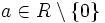$a \in R \setminus \{ 0 \}$,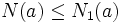$N(a) \le N_1(a)$.
• The elements of norm zero are precisely the units and the elements of norm one are precisely the universal side divisors.
•$N$ is a multiplicatively monotone Euclidean norm, i.e., it is multiplicatively monotone: if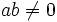$ab \ne 0$,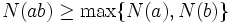$N(ab) \ge \max \{ N(a), N(b) \}$.
•$N$ is an automorphism-invariant Euclidean norm.

Proof

Given: A commutative unital ring$R$ with a Euclidean norm$N_1$.

Construction of the sloewst growing Euclidean norm

We define the norm inductively as follows.

For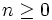$n \ge 0$, define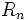$R_n$ as follows:

•$R_0$ is the set of units.
• For$n \ge 1$,$R_n$ is defined as the set of those$x$ such that$x \ne 0$,$x \notin \bigcup_{m=0}^{n-1} R_m$, and for every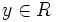$y \in R$, there exists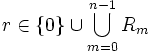$r \in \{ 0 \} \cup \bigcup_{m=0}^{n-1} R_m$ such that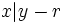$x | y - r$.

For any$x \in R \setminus \{ 0 \}$,$N(x)$ is defined as the unique$n$ such that$x \in R_n$.

Note that$N(x)$ is well-defined for all$x$,$N$ is Euclidean by definition: for every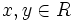$x,y \in R$ with$x \ne 0$, there exists$r$ such that$x | y -r$ with$r = 0$ or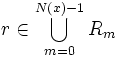$r \in \bigcup_{m=0}^{N(x)-1} R_m$, which is precisely saying that$r = 0$ or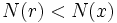$N(r) < N(x)$. Thus, we can write: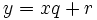$y = xq + r$

with$r = 0$ or$N(r) < N(x)$.

Proof that this Euclidean norm exists for a Euclidean ring and is smaller than any other Euclidean norm

We prove that if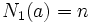$N_1(a) = n$ for the Euclidean norm$N_1$, then$a \in \bigcup_{m=0}^n R_m$. This will prove that$N$ is well-defined and$N(x) \le N_1(x)$ for all nonzero$x$.

Given:$a \in R \setminus \{ 0 \}$ with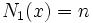$N_1(x) = n$.

To prove:$a \in \bigcup_{m=0}^n R_m$.

Proof: We prove this claim by induction on$n$.

Base case: When$n = 0$, we have$N_1(x) = 0$. By fact (1),$a$ is a unit, so$a \in R_0$ by definition.

Induction step: Suppose$n > 1$, and the statement is true for$0 \le m \le n - 1$. We have$N_1(x) = n$.

• If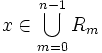$x \in \bigcup_{m=0}^{n-1} R_m$, we are done.
• Suppose$x \notin \bigcup_{m=0}^{n-1} R_m$. For any$y \in R$, the division algorithm yields:$y = xq + r$

where$r = 0$ or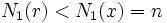$N_1(r) < N_1(x) = n$. By the inductive assumption, $0 \} \cup \bigcup_{m=0}^n-1$ . Thus, for any$y \in R$,$x | y - r$ for some$r \in \{ 0 \} \cup \bigcup_{m=0}^{n-1} R_m$.

Thus,$x \in R_n$.

Proof that the norm is multiplicatively monotone

Given: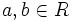$a,b \in R$ such that$ab \ne 0$.

To prove:$N(ab) \ge \max \{ N(a), N(b) \}$.

Proof: One way of proving this is to invoke fact (2), which states that given any Euclidean norm, we can obtain a smaller multiplicatively monotone Euclidean norm. Since the norm$N$ is the smallest Euclidean norm, it must be equal to the multiplicatively monotone Euclidean norm obtained from it using fact (2).

We can also check the condition directly. If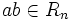$ab \in R_n$, then either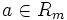$a \in R_m$ for some$m < n$, or$a$ satisfies all the conditions for being in$R_n$. In either case,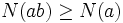$N(ab) \ge N(a)$. Similarly,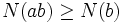$N(ab) \ge N(b)$.

Proof that the norm is automorphism-invariant

The way the norm is defined is clearly automorphism-invariant, since it depends on no choices.